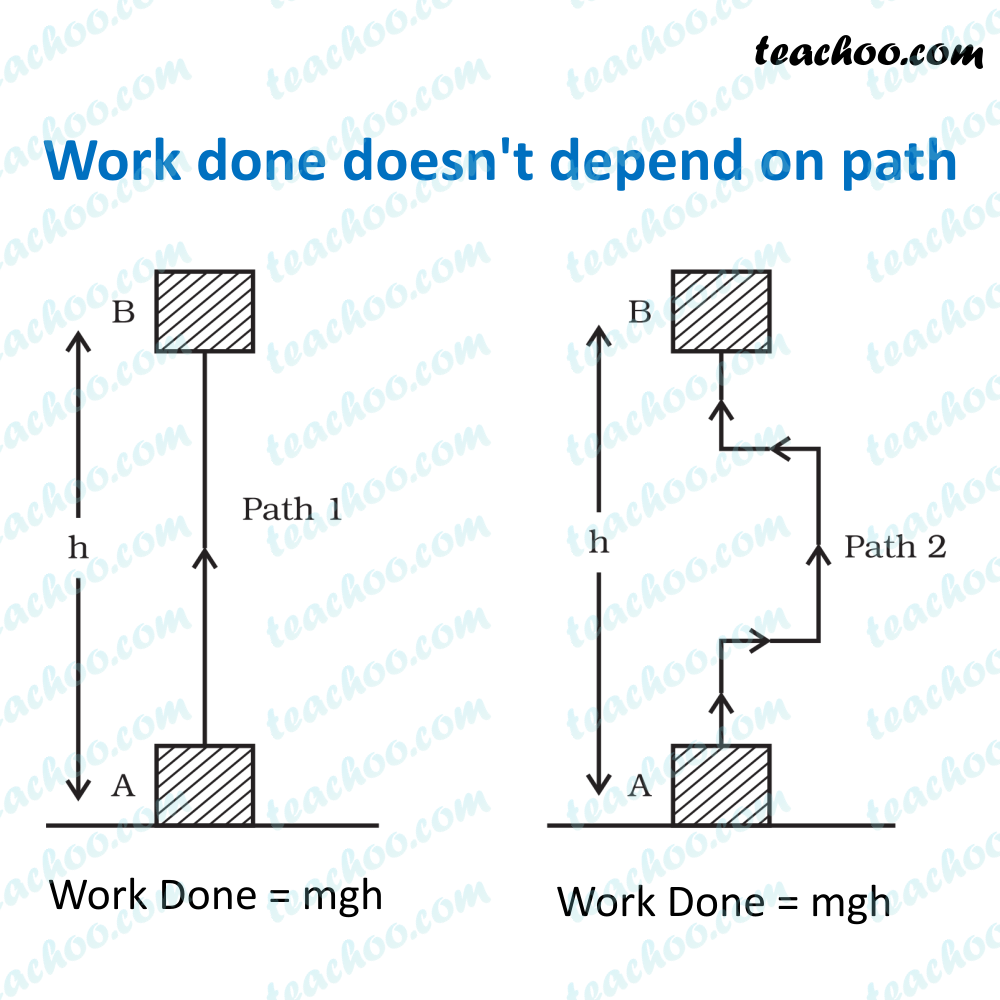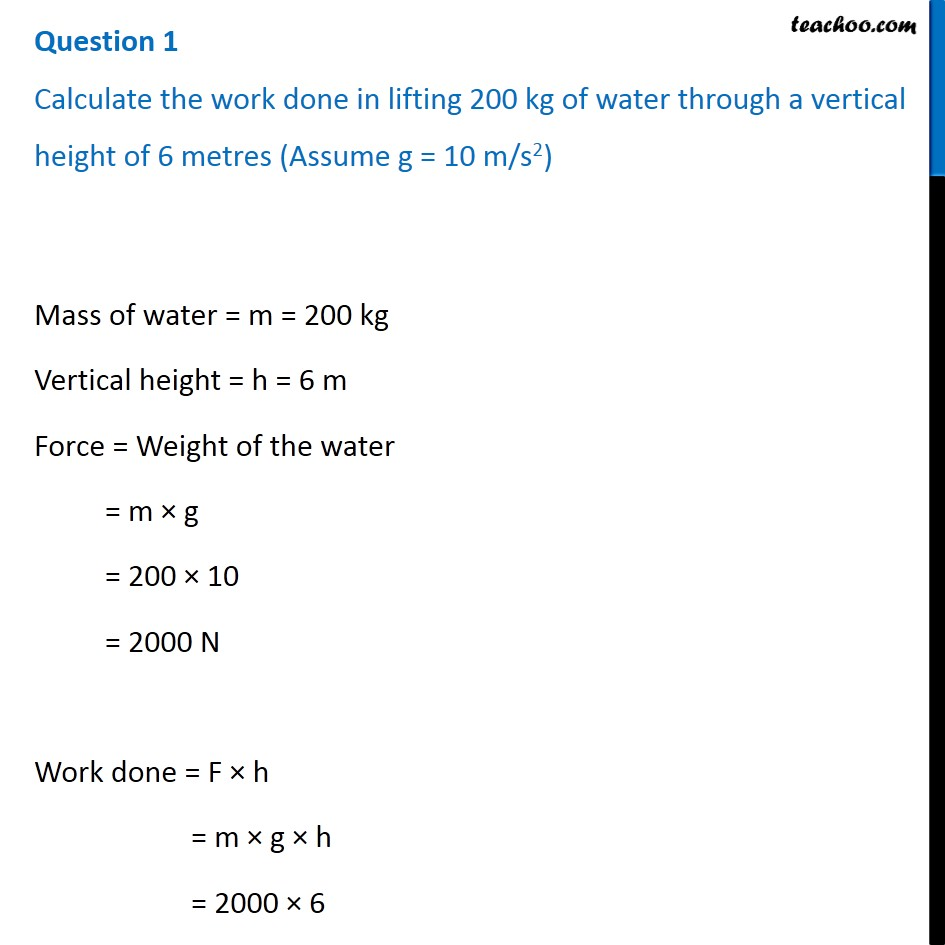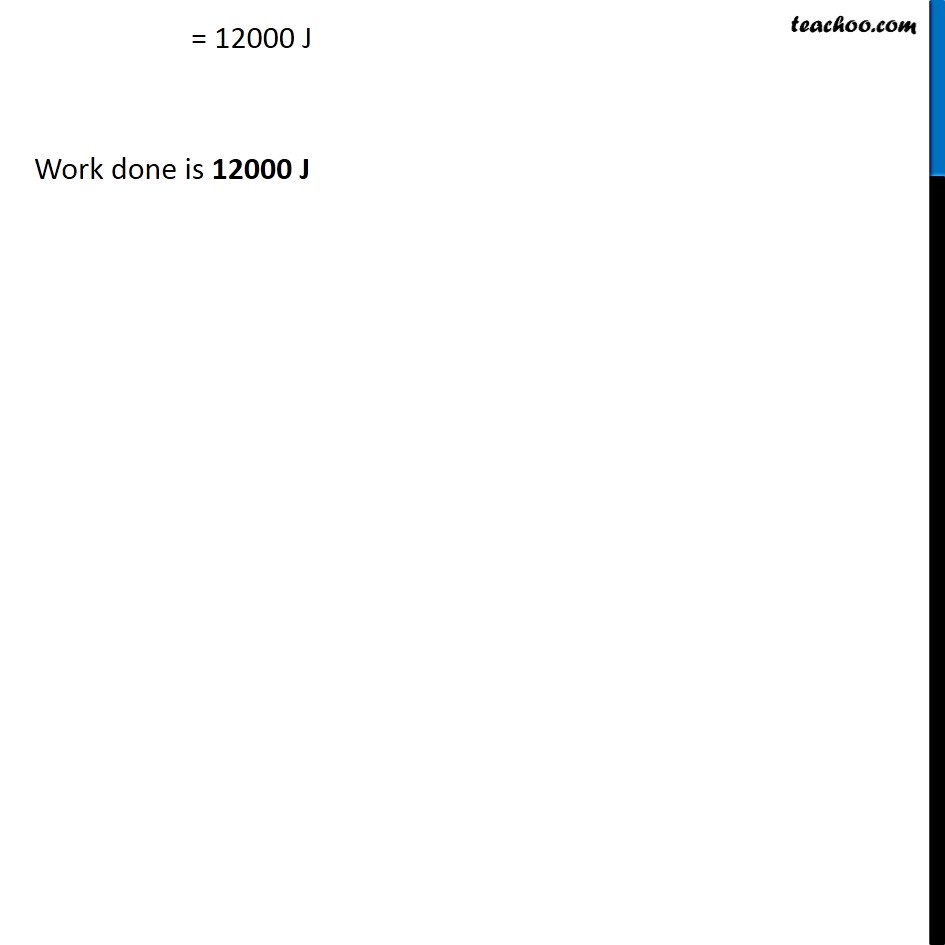Concepts

Class 9
Chapter 11 Class 9 - Work and Energy

We know that

Gravity of earth attracts all objects towards earth

Example -

A ball falls towards ground because of force of gravity of earth

But if we pick up the ball, we are actually working against gravity

Hence, we can say that

Work done in lifitng a body is work done against gravity

## How to Calculate Work Done Against Gravity

We know that

Work = Force × Distance

### Calculating Force

Here Force is Gravitational force

We know that

Force = Mass × Acceleration

Gravitational Force = Mass × Acceleration due to Gravity

F = m × g

### Calculating Distance

We know that Distance in case of body falling to earth/from earth is Height of Object

So in this case

Distance = Height

s = h

Now we know that

Work = Force × Distance

W = F × s

W = m × g × h

## Height does not depend on path of the object.

Work Done = m × g × h

Here, height is measured from initial and final points, it does not depend on path travelled by the object##(adsbygoogle = window.adsbygoogle || []).push({});Learn in your speed, with individual attention - Teachoo Maths 1-on-1 Class

### Transcript

Question 1 Calculate the work done in lifting 200 kg of water through a vertical height of 6 metres (Assume g = 10 m/s2) Mass of water = m = 200 kg Vertical height = h = 6 m Force = Weight of the water = m × g = 200 × 10 = 2000 N Work done = F × h = m × g × h = 2000 × 6 = 12000 J Work done is 12000 J